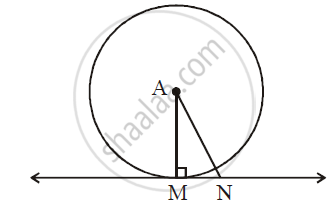# In the Following Figure, Point ‘A’ is the Centre of the Circle. Line Mn is Tangent at Point M. If an = 10 Cm and Mn = 5 Cm, Determine Radius of the Circle. - Geometry

In the following figure, point ‘A’ is the centre of the circle. Line MN is tangent at point M. If AN = 10 cm and MN = 5 cm, determine radius of the circle.#### Solution

Seg AM is the radius and line MN is the tangent to the circle at the point M.

:. angleAMN = 90^@     .....(Tangent is perpendicular to the radius)

In right-angled triangle AMN , by Pythagoras’ theorem

AN^2 = AM^2 + MN^2

:.(10)^2 =AM^2 + (5)^2

:. AM^2 = (10)^2 - (5)^2 = 100 - 25 = 75

:.AM = sqrt75 = sqrt(25xx3)

:.AM = 5sqrt3cm

The radius of the circle is 5sqrt3cm.

Concept: Theorem - Converse of Tangent at Any Point to the Circle is Perpendicular to the Radius
Is there an error in this question or solution?